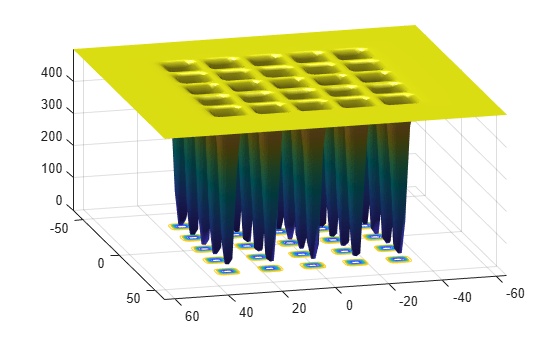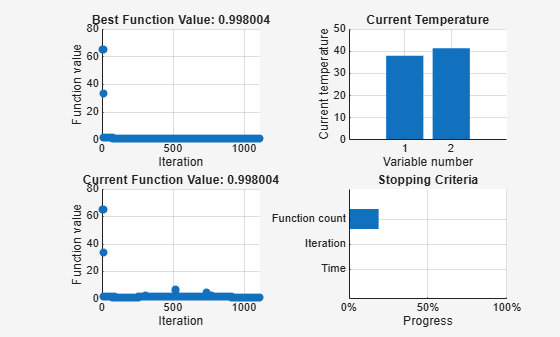Documentation

## Simulated Annealing Options

This example shows how to create and manage options for the simulated annealing function `simulannealbnd` using `optimoptions` in the Global Optimization Toolbox.

### Optimization Problem Setup

`simulannealbnd` searches for a minimum of a function using simulated annealing. For this example we use `simulannealbnd` to minimize the objective function `dejong5fcn`. This function is a real valued function of two variables and has many local minima making it difficult to optimize. There is only one global minimum at `x =(-32,-32)`, where `f(x) = 0.998`. To define our problem, we must define the objective function, start point, and bounds specified by the range `-64 <= x(i) <= 64` for each `x(i)`.

```ObjectiveFunction = @dejong5fcn; startingPoint = [-30 0]; lb = [-64 -64]; ub = [64 64];```

The function `plotobjective` in the toolbox plots the objective function over the range `-64 <= x1 <= 64`, `-64 <= x2 <= 64`.

```plotobjective(ObjectiveFunction,[-64 64; -64 64]); view(-15,150);```Now, we can run the `simulannealbnd` solver to minimize our objective function.

```rng default % For reproducibility [x,fval,exitFlag,output] = simulannealbnd(ObjectiveFunction,startingPoint,lb,ub);```
```Optimization terminated: change in best function value less than options.FunctionTolerance. ```
`fprintf('The number of iterations was : %d\n', output.iterations);`
```The number of iterations was : 1095 ```
`fprintf('The number of function evaluations was : %d\n', output.funccount);`
```The number of function evaluations was : 1104 ```
`fprintf('The best function value found was : %g\n', fval);`
```The best function value found was : 2.98211 ```

Note that when you run this example, your results may be different from the results shown above because simulated annealing algorithm uses random numbers to generate points.

`simulannealbnd` can accept one or more plot functions through an 'options' argument. This feature is useful for visualizing the performance of the solver at run time. Plot functions are selected using `optimoptions`. The toolbox contains a set of plot functions to choose from, or you can provide your own custom plot functions.

To select multiple plot functions, set the `PlotFcn` option via the `optimoptions` function. For this example, we select `saplotbestf`, which plots the best function value every iteration, `saplottemperature`, which shows the current temperature in each dimension at every iteration, `saplotf`, which shows the current function value (remember that the current value is not necessarily the best one), and `saplotstopping`, which plots the percentage of stopping criteria satisfied every ten iterations.

```options = optimoptions(@simulannealbnd, ... 'PlotFcn',{@saplotbestf,@saplottemperature,@saplotf,@saplotstopping});```

Run the solver.

`simulannealbnd(ObjectiveFunction,startingPoint,lb,ub,options);````Optimization terminated: change in best function value less than options.FunctionTolerance. ```

### Specifying Temperature Options

The temperature parameter used in simulated annealing controls the overall search results. The temperature for each dimension is used to limit the extent of search in that dimension. The toolbox lets you specify initial temperature as well as ways to update temperature during the solution process. The two temperature-related options are the `InitialTemperature` and the `TemperatureFcn`.

Specifying initial temperature

The default initial temperature is set to 100 for each dimension. If you want the initial temperature to be different in different dimensions then you must specify a vector of temperatures. This may be necessary in cases when problem is scaled differently in each dimensions. For example,

`options = optimoptions(@simulannealbnd,'InitialTemperature',[300 50]);`

`InitialTemperature` can be set to a vector of length less than the number of variables (dimension); the solver expands the vector to the remaining dimensions by taking the last element of the initial temperature vector. Here we want the initial temperature to be the same in all dimensions so we need only specify the single temperature.

`options.InitialTemperature = 100;`

Specifying a temperature function

The default temperature function used by `simulannealbnd` is called `temperatureexp`. In the temperatureexp schedule, the temperature at any given step is .95 times the temperature at the previous step. This causes the temperature to go down slowly at first but ultimately get cooler faster than other schemes. If another scheme is desired, e.g. Boltzmann schedule or "Fast" schedule annealing, then `temperatureboltz` or `temperaturefast` can be used respectively. To select the fast temperature schedule, we can update our previously created options, changing `TemperatureFcn` directly.

`options.TemperatureFcn = @temperaturefast;`

Specifying reannealing

Reannealing is a part of annealing process. After a certain number of new points are accepted, the temperature is raised to a higher value in hope to restart the search and move out of a local minima. Performing reannealing too soon may not help the solver identify a minimum, so a relatively high interval is a good choice. The interval at which reannealing happens can be set using the `ReannealInterval` option. Here, we reduce the default reannealing interval to 50 because the function seems to be flat in many regions and solver might get stuck rapidly.

`options.ReannealInterval = 50;`

Now that we have setup the new temperature options we run the solver again

`[x,fval,exitFlag,output] = simulannealbnd(ObjectiveFunction,startingPoint,lb,ub,options);`
```Optimization terminated: change in best function value less than options.FunctionTolerance. ```
`fprintf('The number of iterations was : %d\n', output.iterations);`
```The number of iterations was : 1306 ```
`fprintf('The number of function evaluations was : %d\n', output.funccount);`
```The number of function evaluations was : 1321 ```
`fprintf('The best function value found was : %g\n', fval);`
```The best function value found was : 16.4409 ```

### Reproducing Results

`simulannealbnd` is a nondeterministic algorithm. This means that running the solver more than once without changing any settings may give different results. This is because `simulannealbnd` utilizes MATLAB® random number generators when it generates subsequent points and also when it determines whether or not to accept new points. Every time a random number is generated the state of the random number generators change.

To see this, two runs of `simulannealbnd` solver yields:

`[x,fval] = simulannealbnd(ObjectiveFunction,startingPoint,lb,ub,options);`
```Optimization terminated: change in best function value less than options.FunctionTolerance. ```
`fprintf('The best function value found was : %g\n', fval);`
```The best function value found was : 1.99203 ```

And,

`[x,fval] = simulannealbnd(ObjectiveFunction,startingPoint,lb,ub,options);`
```Optimization terminated: change in best function value less than options.FunctionTolerance. ```
`fprintf('The best function value found was : %g\n', fval);`
```The best function value found was : 10.7632 ```

In the previous two runs `simulannealbnd` gives different results.

We can reproduce our results if we reset the states of the random number generators between runs of the solver by using information returned by `simulannealbnd`. `simulannealbnd` returns the states of the random number generators at the time `simulannealbnd` is called in the output argument. This information can be used to reset the states. Here we reset the states between runs using this output information so the results of the next two runs are the same.

`[x,fval,exitFlag,output] = simulannealbnd(ObjectiveFunction,startingPoint,lb,ub,options);`
```Optimization terminated: change in best function value less than options.FunctionTolerance. ```
`fprintf('The best function value found was : %g\n', fval);`
```The best function value found was : 20.1535 ```

We reset the state of the random number generator.

```strm = RandStream.getGlobalStream; strm.State = output.rngstate.State;```

Now, let's run `simulannealbnd` again.

`[x,fval] = simulannealbnd(ObjectiveFunction,startingPoint,lb,ub,options);`
```Optimization terminated: change in best function value less than options.FunctionTolerance. ```
`fprintf('The best function value found was : %g\n', fval);`
```The best function value found was : 20.1535 ```

### Modifying the Stopping Criteria

`simulannealbnd` uses six different criteria to determine when to stop the solver. `simulannealbnd` stops when the maximum number of iterations or function evaluation is exceeded; by default the maximum number of iterations is set to Inf and the maximum number of function evaluations is `3000*numberOfVariables`. `simulannealbnd` keeps track of the average change in the function value for `MaxStallIterations` iterations. If the average change is smaller than the function tolerance, `FunctionTolerance`, then the algorithm will stop. The solver will also stop when the objective function value reaches `ObjectiveLimit`. Finally the solver will stop after running for `MaxTime` seconds. Here we set the `FunctionTolerance` to 1e-5.

`options.FunctionTolerance = 1e-5;`

Run the `simulannealbnd` solver.

`[x,fval,exitFlag,output] = simulannealbnd(ObjectiveFunction,startingPoint,lb,ub,options);`
```Optimization terminated: change in best function value less than options.FunctionTolerance. ```
`fprintf('The number of iterations was : %d\n', output.iterations);`
```The number of iterations was : 1843 ```
`fprintf('The number of function evaluations was : %d\n', output.funccount);`
```The number of function evaluations was : 1864 ```
`fprintf('The best function value found was : %g\n', fval);`
```The best function value found was : 6.90334 ```

Watch now